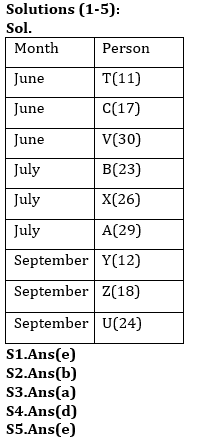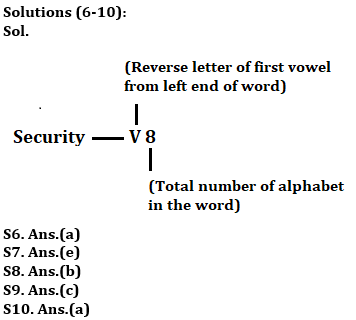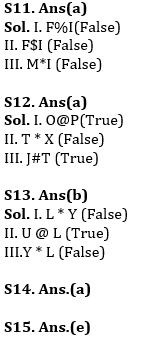Latest Banking jobs   »   Reasoning Ability Quiz For IBPS PO...

# Reasoning Ability Quiz For IBPS PO Mains 2022- 11th January

Directions (1-5): Study the information carefully and answer the questions given below.
There are nine persons i.e. T, U, V, X, Y, Z,A, B and C are born on different dates in three different months i.e. June, July and September but not necessarily in the same order. Three persons born in any of the month. The one who born on 11th born before the one who born on 17th. One person born between U and Y. Both U and Y does not born in the month having odd number of days. Four persons born between Y and C. The one who born immediately after C was born on 30th. X was born on 26th but not in month C was born. B born on 23rd and immediately before X but not in month which has even number of days. The one who born before A born on 11th. The number of persons born between T and B is same as the number of persons born between A and V. Z was born on 18th of the month. Birthdate of U is immediate next to that of B. Only one person born between X and V but both were not born in same month. The one who born immediately before Y was born on 29th. One of the persons born on 17th in the month of June. There are as many days between birthdays of Y and Z as between Z and U.

Q1. What is the birthdate of T?
(a) 17
(b) 26
(c) 12
(d) 29
(e) None of these

Q2. How many persons born between Z and C?
(a) Two
(b) Five
(c) Four
(d) One
(e) None

Q3. Who among the following was born immediate before B?
(a) The one who born on 30th
(b) C
(c) Z
(d) The one who born on 26th
(e) None of these

Q4. Which of the following pair of persons born in July month?
(a) A, T, V
(b) T, U, X
(c) B, U, C
(d) B, X, A
(e) None of these

Q5. How many persons are younger than B?
(a) Four
(b) One
(c) Three
(d) Two
(e) More than Four

Directions (6-10): Study the information and answer the following questions:
In a certain code language
“Security under Hack” is coded as ” Z4 V8 F5 ”
“Common incident Study” is coded as “F5 R8 L6″
“Attention Perfect Game” is coded as “Z9 V7 Z4”

Q6. What is the code for ‘Supported’?
(a) F9
(b) Z9
(c) R8
(d) F8
(e) None of these

Q7. What is the code for ‘Asking’?
(a) R6
(b) K7
(c) T6
(d) H7
(e) None of these

Q8. What is the code for ‘Concern’?
(a) X7
(b) L7
(c) X6
(d) I7
(e) None of these

Q9. What is the code for ‘Strategy’?
(a) H8
(b) G6
(c) Z8
(d) G9
(e) None of these

Q10. What is the code for ‘Thirty’?
(a) R6
(b) B6
(c) R7
(d) G6
(e) None of these

Directions (11-13): In the following questions, the symbols @, #, %, \$ and © are used with the following meaning as illustrated below-
‘P@Q’ means ‘P is neither greater than nor equal to Q’
‘P*Q’ means ‘P is neither equal to nor smaller than Q’
‘P\$Q’ means ‘P is neither smaller than nor greater than Q’
‘P%Q’ means ‘P is not smaller than Q’
‘P#Q’ means ‘P is not greater than Q’
Now in each of the following questions assuming the given statement to be true, find which of the three conclusions I, II and III given below them is/are definitely true and give your answer accordingly.

Q11. Statements: F*G%H\$I*J#K\$L@M#N
Conclusions: I. F%I
II. F\$I
III. M*I
(a) None is true
(b) Only I is true
(c) Only III is true
(d) Either I or II are true
(e) None of these

Q12. Statements: Y*T%O\$J@P*X%Z
Conclusions: I. O@P
II. T * X
III. J#T
(a) Only I and III are true
(b) Only II and III are true
(c) Only I and II are true
(d) All are true
(e) None of these

Q13. Statements: L \$ P, P * U, U @ M, Y * U
Conclusions: I. L * Y
II. U @ L
III.Y * L
(a) None is true
(b) Only II is true
(c) Only I and II are true
(d) Only II and III are true
(e) All are true

Q14. Which of the following symbols should replace the sign (@) and (%) respectively in the given expression in order to make the expression E ≥ T and S> A definitely true?
E ≥ I ≥ R = W = A @ T ≤ W % S
(a) =, <
(b) ≥, <
(c) >, ≤
(d) ≤, =
(e) ≤, ≤

Q15. Which of the following will be definitely true if the given expression E=R<V>S>M=O>X=Y>T is definitely true?
(a) V < T
(b) X > E
(c) R > M
(d) Y ≥ R
(e) Y < V

Solutions#### Congratulations!Union Budget 2023-24: Free PDF